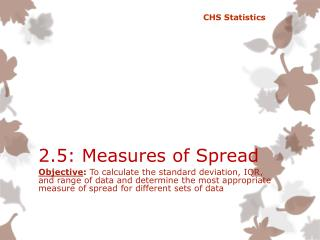DownloadDownload PresentationTélécharger la présentation- - - - - - - - - - - - - - - - - - - - - - - - - - - E N D - - - - - - - - - - - - - - - - - - - - - - - - - - -
##### Presentation Transcript

1. CHS Statistics 2.5: Measures of Spread Objective: To calculate the standard deviation, IQR, and range of data and determine the most appropriate measure of spread for different sets of data

2. Warm-Up • You are trying to decide which Biology class to take. You have 2 instructors to choose from. Professor Smith’s classes have a mean score of 82% with scores varying from 60% to 95%. Professor Jones’ classes have a mean score of 85% with scores varying from 78% to 90%. Who would you choose? • You are trying to figure out which brand of light bulbs to buy. Both have a mean lifetime of 1100 hours. Which would you buy? Given this scenario you may purchase the cheaper of the two. However, if you knew the GE bulb’s lifetime varies from 500 to 1500 hours and the Walmart brand bulb’s lifetime varies from 1000 to 1200 hours would you still buy the cheaper one?

3. 4 Measures of Variation (Spread) • Range • Interquartile Range • Variance • Standard Deviation

4. 4 Measures of Variation (Spread) • Range – the distance between the maximum and minimum values Range = Max Value – Min Value • Example: 1 2 5 8 12 16 20 • Example: 1 18 19 20 20 20 20 Affected by outliers?

5. 4 Measures of Variation (Spread) • Interquartile Range – The distance between the first and third quartiles IQR = Q3 – Q1 • Contains the middle 50% of data Q1 – One quarter (25%) of the data lie below the first quartile Q2 – the median Q3 – one quarter (25%) of the data lie above the third quartile

6. 4 Measures of Variation (Spread) • Interquartile Range • Example: Find the IQR of the set of data: 1 18 19 20 20 20 20 Affected by outliers?

7. 4 Measures of Variation (Spread) • Interquartile Range • You can find the quartiles in the graphing calculator just like you would find the mean. Scroll down and find Q1 and Q3. • Enter your data into L1 (Stat  Edit) • To clear data, be sure to highlight the title (L1) and press CLEAR  Enter (DO NOT PRESS DELETE) • Once your data is entered, press STAT  CALC  1-Variable Stat

8. 4 Measures of Variation (Spread) • Variance • The variance, notated by s2, is found by summing the squared deviations and (almost) averaging them: • Why are we ALMOST averaging them? • The variance will play a role later in our study, but it is problematic as a measure of spread—it is measured in squared units! Population Variance: Sample Variance:

9. 4 Measures of Variation (Spread) • Standard Deviation - the square root of the variance and is measured in the same units as the original data • The standard deviation measures how far each value is from the mean. • Unless otherwise specified, assume the data collected are a sample and find the sample SD. Sample Standard Deviation: Population Standard Deviation:

10. 4 Measures of Variation (Spread) • Standard Deviation Steps for Calculation: • Find the sample mean. • Subtract the sample mean from each value of data. • Square the difference from #2. • Find the sum of the values from #2. • Plug into the formula. Affected by outliers?

11. 4 Measures of Variation (Spread) • Standard Deviation Example: Points scored Week 1 NFL Winners.

12. 4 Measures of Variation (Spread) • Standard Deviation • Steps on the calculator: Enter your data into L1 (Stat  Edit) • To clear data, be sure to highlight the title (L1) and press CLEAR  Enter (DO NOT PRESS DELETE) • Once your data is entered, press STAT  CALC  1-Variable Stat • Use sx for the sample standard deviation and for population standard deviation.

13. Rule of Thumb for Spread Look at a histogram of your data to know the best measure of spread to report! • When the histogram of your data is fairly symmetric, report the standard deviation, because it is a more accurate measure of spread. • When the histogram of your data is skewed in any direction, report the IQR as a more appropriate measure of spread. Why?

14. Assignment 2.5 Worksheet